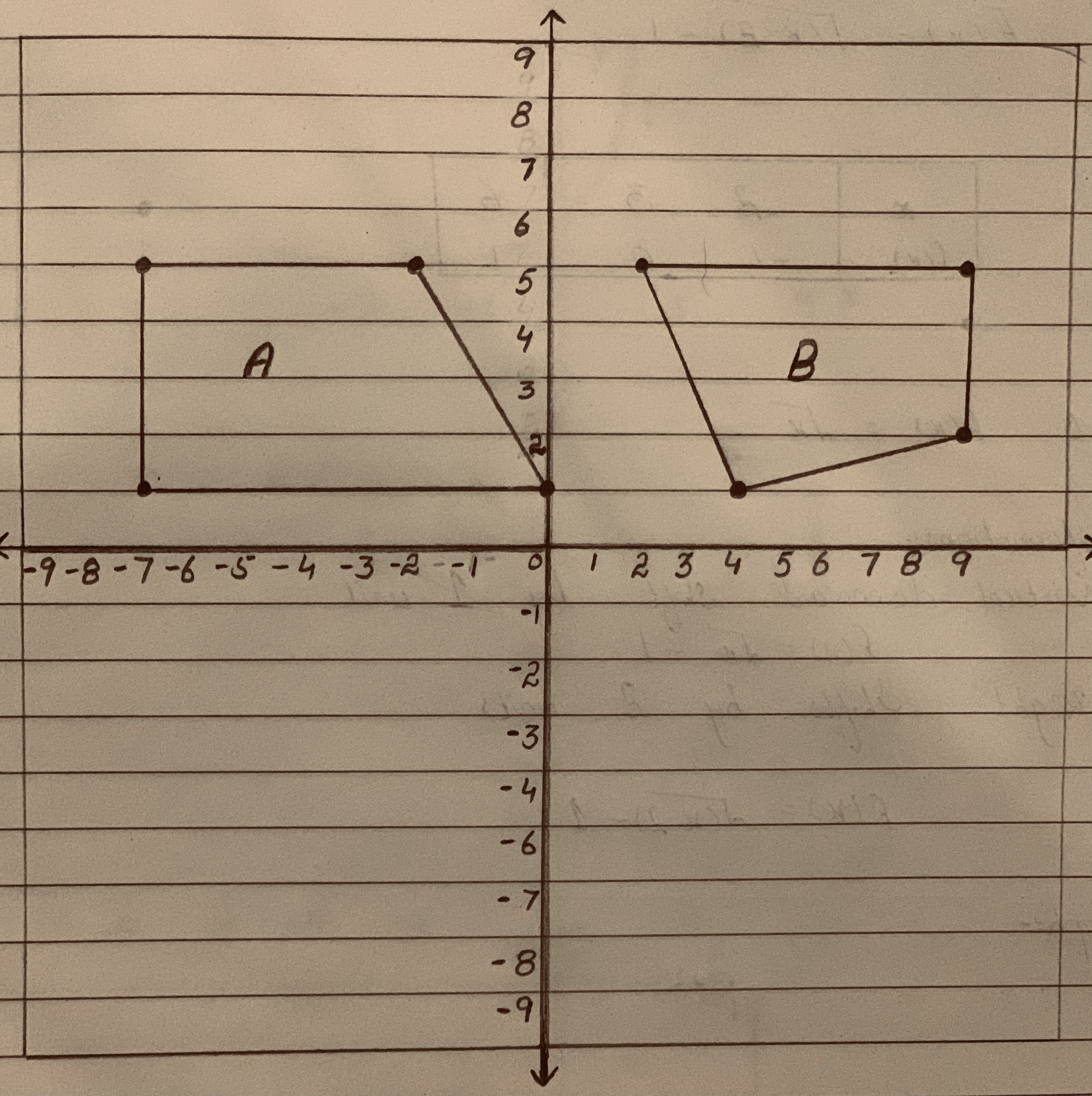# Describe a sequence of translations that can be used to show that Figures A and A' are congruent and that Figures B and B' are congurent. Show your work and explaic your work reasoning.Question
SequencesDescribe a sequence of translations that can be used to show that Figures A and A' are congruent and that Figures B and B' are congurent. Show your work and explaic your work reasoning.2021-02-01
$$\displaystyle{A}\rightarrow{A}'$$
Translate A up by 5 units and then to the right by 4 units. Doing so, A will overlap with A' and you'll be able to see that they are congruent.
$$\displaystyle{B}\rightarrow{B}'$$
Translate B up by 2 units and then to the right by 6 units. Doing so, B will overlap with B' and you'll be able to see that they are congruent.

### Relevant QuestionsSeveral terms of a sequence {a_n}_(n=1)^oo are given. a. Find the next two terms of the sequence. b. Find a recurrence relation that generates the sequence (supply the initial value of the index and the first term of the sequence). c. Find an explicit formula for the nth term of the sequence. {1, 3, 9, 27, 81, ...}Lily wants to define a transformation (or series of transformations) using only rotations, reflections or translations that takes Figure A to Figure B.Which statement about the transformation that Lily wants to define is true?
A. It can be defined with two reflections.
B.It can be defined with one rotation and one translation.
C. It cannot be defined because Figure A and Figure B are not congruent.
D.It cannot be defined because the longest side of Figure B is on the bottom.Find a recurrence relation for the number of sequences of yellow and purple stones of length N, where each sequence has the property that no two adjacent stones are purple.The first of an arithmetic sequence is 2 and has the property that its second, third, and seventh terms are consecutive terms of a geometric sequence.Write a recursive rule and an explicit rule for an arithmetic sequence that models a situation. Then use the rule to answer the question. Jack begins an exercise routine for 10 minutes each day. Each week he plans to add 10 minutes per day to his exercise routine. For how many minutes will he exercise for each day on the 7th week?A soup kitchen makes 16 gallons of soup. Each day, a quarter of the soup is served and the rest is saved for the next day. Write the first five terms of the sequence of the number of fluid ounces of soup left each day. Write an equation that represents the nth term of the sequence. When is all the soup gone?For Questions 1 — 2, use the following. Scooters are often used in European and Asian cities because of their ability to negotiate crowded city streets. The number of scooters (in thousands) sold each year in India can be approximated by the function $$N = 61.86t^2 — 237.43t + 943.51$$ where f is the number of years since 1990. 1. Find the vertical intercept. What is the practical meaning of the vertical intercept in this situation? 2. Use a numerical method to find the year when the number of scooters sold reaches 1 million. (Note that 1 million is 1,000 thousand, so N = 1000) Show three rows of the table you used to support your answer and write a clear answer to the problem.